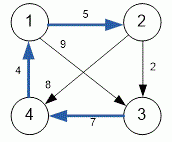Problems

# Floyd

Time limit 1 second
Memory limit 128 MiB

Given a directed weighted graph. Find a pair of vertices, the shortest distance from one of them to another is maximum among all pairs of vertices.

## Input data

The first line contains the number of vertices n (1n100). The next n lines of n numbers describe the weighted matrix. Here -1 means no edges between vertices, and any non-negative number - the presence of an edge of given weight. All elements on the main diagonal are always zero.

## Output data

Print the desired maximum shortest distance.## Examples

Input example #1
4
0 5 9 -1
-1 0 2 8
-1 -1 0 7
4 -1 -1 0

Output example #1
16